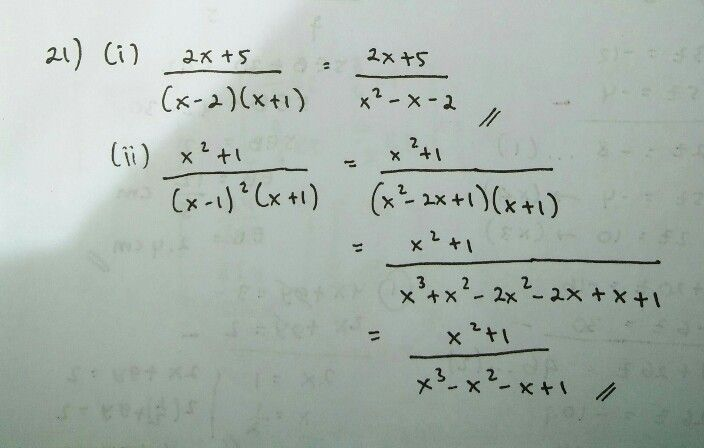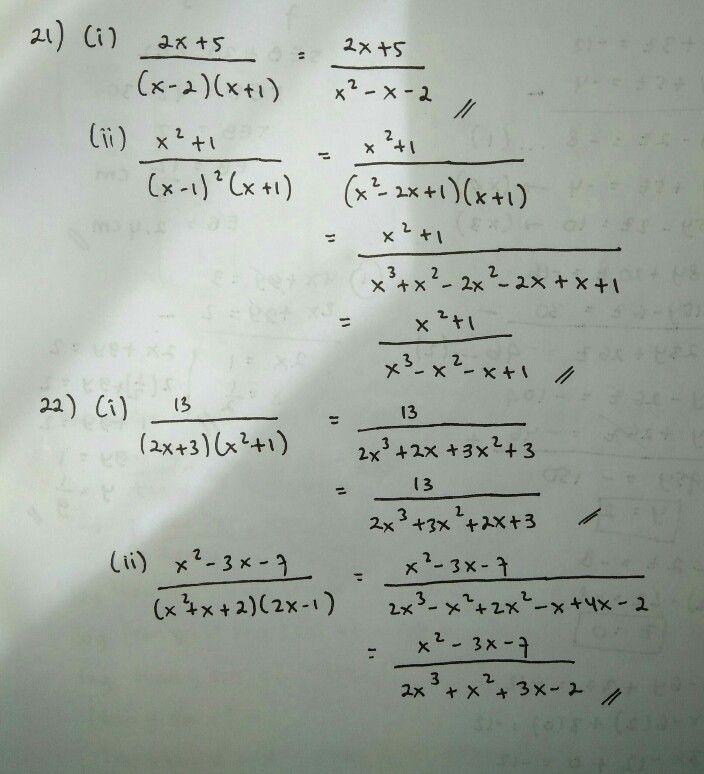Symbol
Problem$21$ Express the following as a sum of partial fractions: $\left(i\right)$ $\dfrac {2x+5} {\left(x-2\right)\left(x+1\right)}$ $\left(ii\right)$ $\dfrac {x^{2}+1} {\left(x-1\right)^{2}\left(x+1\right)}$ $22$ Express the following as a sum of partial fractions: $\left(1\right)$ $\dfrac {13} {\left(2x+3\right)\left(x^{2}+1\right)}$ $\left(ii\right)$ $\dfrac {x^{2}-3x-7} {\left(x^{2}+x+2\right)\left(2x-1}\right)$
Algebra
SolutionQanda teacher - Farah